Mathematical and Physical Journal
for High Schools
Issued by the MATFUND Foundation
 Already signed up? New to KöMaL?

# KöMaL Problems in Physics, February 2013

Show/hide problems of signs:## Problems with sign 'M'

Deadline expired on March 11, 2013.

M. 330. Investigate how balls of different radii, and possibly made of the same material (for example glass or bearing balls), roll along slopes which are covered by some soft, elastic material like foam rubber. Determine the percentage value of the loss in the kinetic energy with measurement.

(6 pont)

statistics## Problems with sign 'P'

Deadline expired on March 11, 2013.

P. 4508. The average speed of the tourists when they walk from the shelter house to the hilltop was 1.5 km/h, whilst on the way back it was 3.5  km/h. The average speed for the whole distance from the shelter house back to the shelter house was 2 km/h. What fraction of the total time of the excursion did the tourist spend on the hilltop having a rest?

(3 pont)

solution (in Hungarian), statistics

P. 4509. How long is the shadow of a 1 m long rod which is fixed perpendicularly to the ground at the equator

a) at noon, on the 21-st of March;

b) 2 hours later after noon on the 21-st of March?

(3 pont)

solution (in Hungarian), statistics

P. 4510. At the end of an initially vertical, hinged rod of negligible mass there is a small heavy sphere. The rod is displaced from its unstable equilibrium position. What is the angular displacement of the rod at the moment when there is no force exerted on the rod?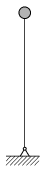(4 pont)

solution (in Hungarian), statistics

P. 4511. A sample of monatomic ideal gas is taken through the process shown in the V-T diagram of the figure. At state A the pressure of the gas is p0, its volume is V0 and its temperature is T0=200 K.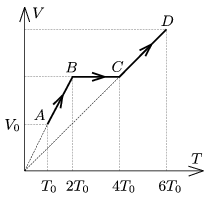a) Draw the graph of the process on the p-V diagram.

b) Find that state E of the process on the p-V diagram for which it is true that the works done by the gas during the processes AE and ED are equal.

c) What is the temperature of the gas at state E?

d) By what factor the absorbed heat in process AE is greater than that of in process ED?

(4 pont)

solution (in Hungarian), statistics

P. 4512. The mass of a plastic slinky is 60 g, its height is 6 cm, and the number of its turns is 40. If it is suspended at one of its end its length is 106 cm. What is the spring constant of one turn of the slinky in N/m?

(4 pont)

solution (in Hungarian), statistics

P. 4513. Two small insulating discs of mass m are placed to the horizontal tabletop, such that initially the distance between their centres is x0=5 cm. A small metal sphere of charge Q=8.10-8 C is fixed at the top of each disc.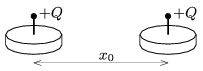One of the discs if fixed to the tabletop and the other is released, and it slides. The coefficient of friction between the disc and the tabletop is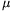=0=0.3. Neglect the electrostatic interaction between the charges and the tabletop.

a) What will the distance x1 be between the centres of the two discs at the moment when the speed of the sliding disc is maximal?

b) What will the distance xm between the two discs be after the sliding discs stops?

c) What is the relationship between x0, x1 and xm?

(5 pont)

solution (in Hungarian), statistics

P. 4514. The readings on the voltmeter and the ammeter connected to the circuit shown in the figure are 10 V and 200 mA, respectively when the switch is open. If the switch is closed the reading on the ammeter increases by 0.1 A, and the voltage across resistor R2 increases by 10 V.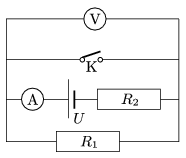a) What are the resistance values of the resistors?

b) What is the voltage of the voltage supply? c) What is the reading on the voltmeter when the switch is closed?

(4 pont)

solution (in Hungarian), statistics

P. 4515. The opposite sides of the vertical conducting frame shown in the figure are parallel, and the top part is connected through a resistor of resistance R=2 ohm. The frame is in uniform magnetic field of B which is perpendicular to the plane of the frame. B=0.8 T. The distance between the parallel, vertical sides of the frame is=20 cm, and the mass of the piece of conductor of negligible resistance, which can slide along the vertical wires without friction is m=200 g. The piece of conductor is released with zero initial speed.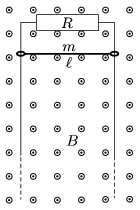a) Graph the net force exerted on the piece of conductor as a function of the speed of the conductor.

b) Estimate how much time elapses while the speed of the conductor changes from 4 m/s to 4.2 m/s. How much distance does it cover during this?

(5 pont)

solution (in Hungarian), statistics

P. 4516. A domestic appliance is connected to the power station by a wire of resistance 12 ohm. The power of the appliance is 1 kW. What is the efficiency of the transmission of electrical energy in case of 230 V, and in case of 10 kV?

(4 pont)

solution (in Hungarian), statistics

P. 4517. Four similar rods of uniform density are connected with frictionless hinges, and this frame is placed to a horizontal smooth tabletop, such that its shape is a square. Vertex P is acted upon by a horizontal force in the direction of the diagonal, and due to this force it begins to move at an acceleration of aP. What is the acceleration of the opposite vertex Q at which it begins to move?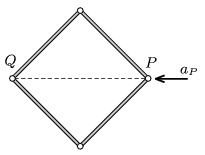(6 pont)

solution (in Hungarian), statistics